# LeetCode打卡 52八皇后Ⅱ&53最大子序和&54螺旋矩阵

LeetCode 47全排列Ⅱ&48旋转图像
LeetCode 49字母异位词分组&50pow(x,n)&51八皇后

### n皇后Ⅱ

n 皇后问题研究的是如何将 n 个皇后放置在 n×n 的棋盘上，并且使皇后彼此之间不能相互攻击。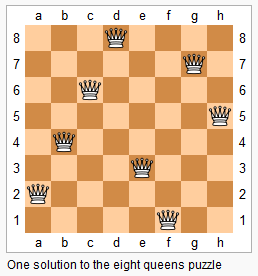[
[".Q..",  // 解法 1
"...Q",
"Q...",
"..Q."],

["..Q.",  // 解法 2
"Q...",
"...Q",
".Q.."]
]


n皇后问题我想跟着我们打卡的铁铁们都应该刷烂了，核心思路就是模拟试探，典型的回溯算法，如果八皇后还不会的请看这篇：回溯算法 | 追忆那些年曾难倒我们的八皇后问题

 boolean shu[];
boolean zuoxie[];
boolean youxie[];
int count=0;
public int totalNQueens(int n) {

shu=new boolean[n];
zuoxie=new boolean[n*2-1];
youxie=new boolean[n*2-1];
dfs(0,n);
return count;

}
//行 map地图

private void dfs(int index,int n) {
// TODO Auto-generated method stub
if(index==n)//存入
{
count++;
}
else {
for(int j=0;j<n;j++)
{
if(!shu[j]&&!zuoxie[index+j]&&!youxie[index+(n-1-j)])
{

shu[j]=true;
zuoxie[index+j]=true;
youxie[index+(n-1-j)]=true;
dfs(index+1, n);
shu[j]=false;
zuoxie[index+j]=false;
youxie[index+(n-1-j)]=false;
}
}
}
}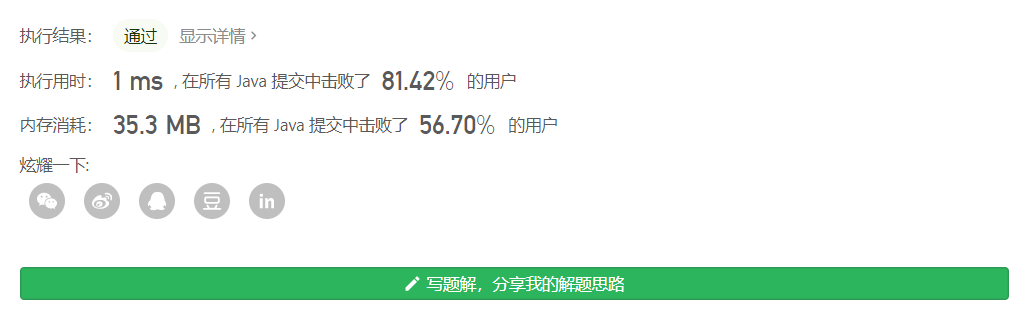## 最大子序列和

dp=a
dp[i]=max(dp[i-1]+a[i],a[i])


public int maxSubArray(int[] nums) {
int dp[]=new int[nums.length];
int max=nums;
dp=nums;
for(int i=1;i<nums.length;i++)
{
dp[i]=Math.max(dp[i-1]+nums[i],nums[i]);
if(dp[i]>max)
max=dp[i];
}
return max;
}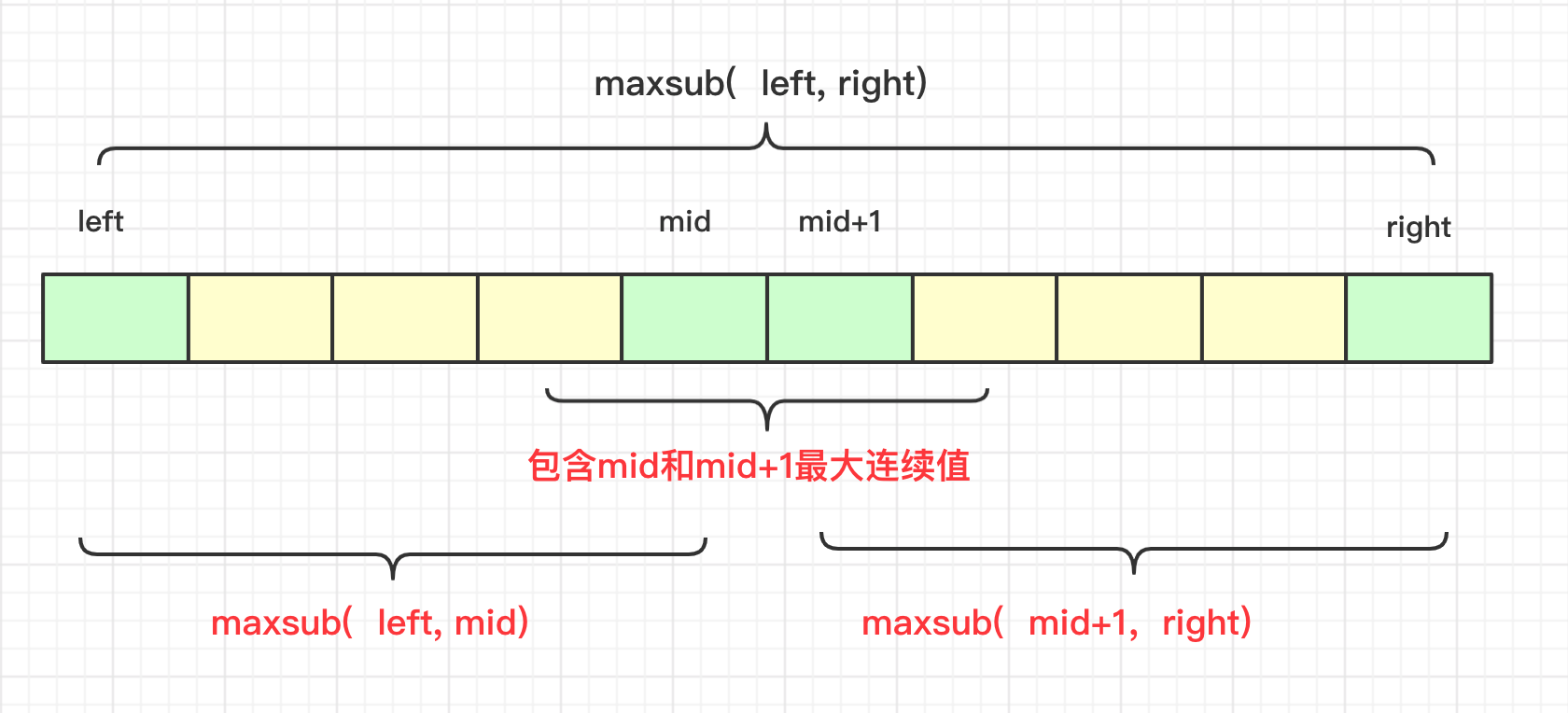public int maxSubArray(int[] nums) {

int max=maxsub(nums,0,nums.length-1);
return max;
}
int maxsub(int nums[],int left,int right)
{
if(left==right)
return  nums[left];
int mid=(left+right)/2;
int leftmax=maxsub(nums,left,mid);
int rightmax=maxsub(nums,mid+1,right);

int midleft=nums[mid];
int midright=nums[mid+1];
int team=0;
for(int i=mid;i>=left;i--)
{
team+=nums[i];
if(team>midleft)
midleft=team;
}
team=0;
for(int i=mid+1;i<=right;i++)
{
team+=nums[i];
if(team>midright)
midright=team;
}
int max=midleft+midright;//中间的最大值
if(max<leftmax)
max=leftmax;
if(max<rightmax)
max=rightmax;
return  max;
}


## 螺旋矩阵

输入:
[
[ 1, 2, 3 ],
[ 4, 5, 6 ],
[ 7, 8, 9 ]
]

[
[1, 2, 3, 4],
[5, 6, 7, 8],
[9,10,11,12]
]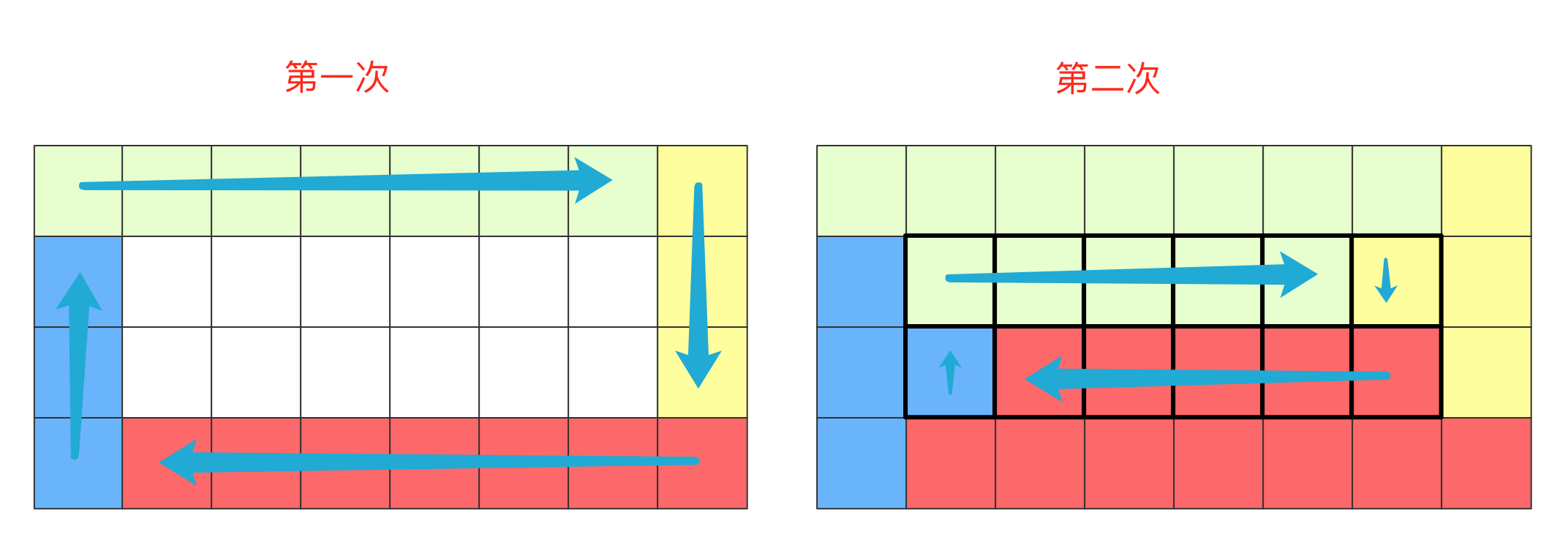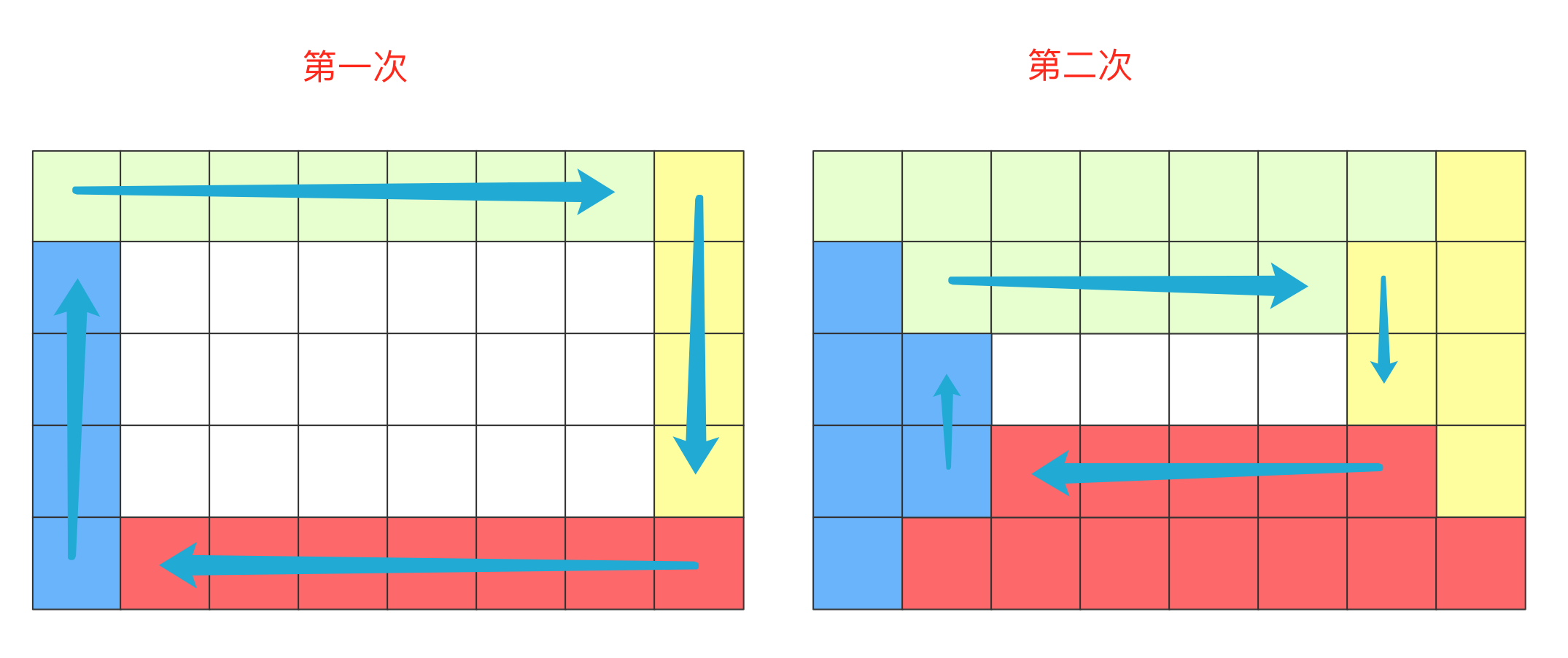public List<Integer> spiralOrder(int[][] matrix) {
List<Integer>list=new ArrayList<Integer>();
if(matrix==null||matrix.length==0)return list;
int m=matrix.length;
int n=matrix.length;
int min=Math.min(m, n);
int index;
for( index=0;index<min/2;index++)
{
for(int i=index;i<n-index-1;i++)//最上面
{
}
for(int i=index;i<m-index-1;i++)
{
}
for(int i=n-index-1;i>index;i--)
{
}
for(int i=m-index-1;i>index;i--)
{
}

}
if(min%2==1)
{
for(int i=index;i<=n-index-1;i++)//最上面
{
}
for(int i=index+1;i<=m-index-1;i++)
{
}
}
return list;
}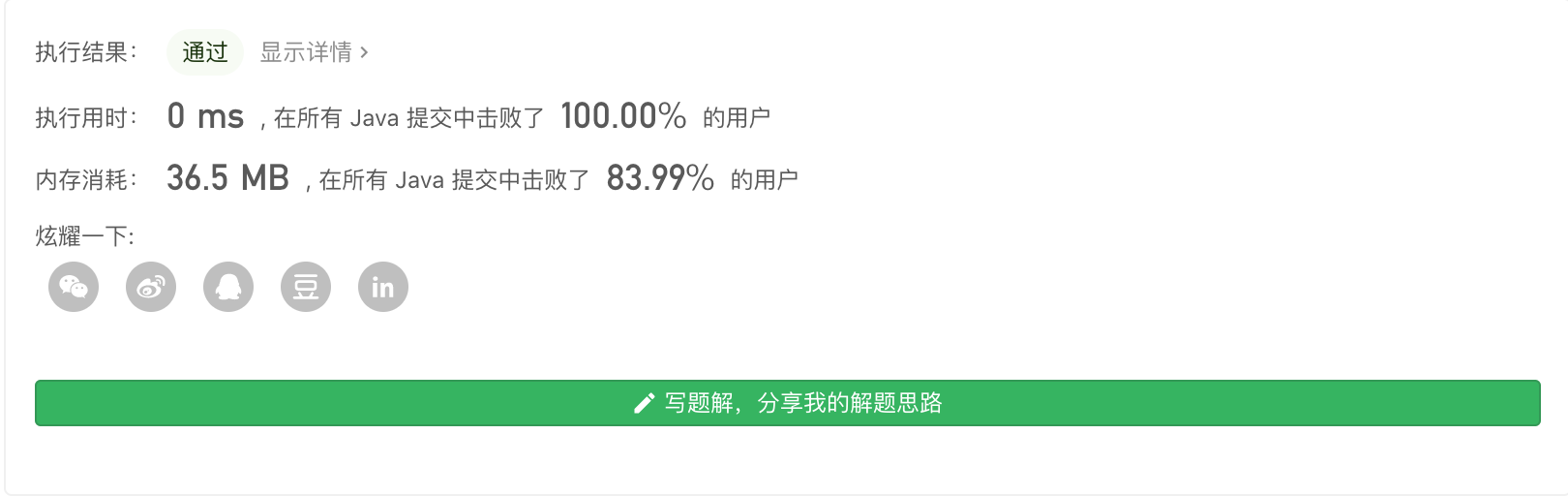Big sai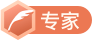CSDN认证博客专家 数据结构与算法 爬虫 Java

06-093419
09-19126602-151万+
09-1342
02-151万+
10-10354
11-14174
11-15158
11-011131
11-08237点击重新获取扫码支付余额充值